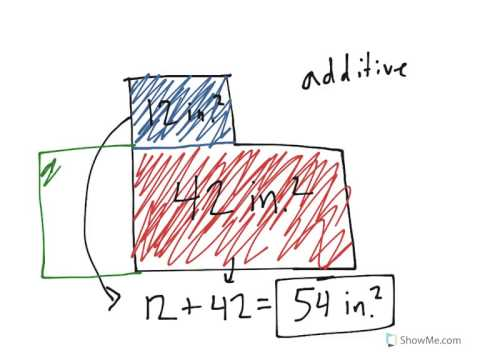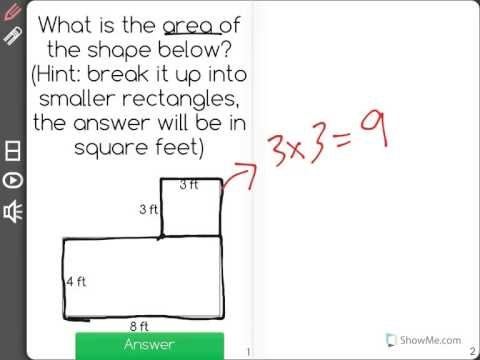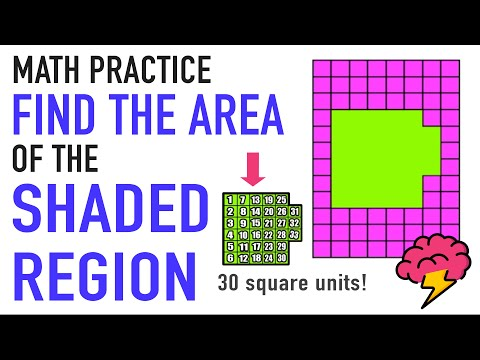# [3.MD.7d-1.0] Adding Partial Areas - Common Core Standard

##By Freckle education

Recognize area as additive# [3.MD.7d-2.0] Adding Partial Areas - Common Core Standard

##By Freckle education

Find areas of rectilinear figures by decomposing them into non-overlapping rectangles and adding the areas of the non-overlapping parts.# How to Find Area of a Region | 3rd Grade Common Core Math

##By Mashup Math

additive where we can divide a region into smaller rectangular shapes, find the area of each, and find the sum.# Acute Triangle - YourTeacher.com - Geometry Help

##By yourteachermathhelp

In this lesson students learn to classify triangles as acute (all angles acute) right (one angle right) or obtuse (one angle obtuse). Students also learn that the sum of the measures of the angles of a triangle is 180 degrees and students are asked to use this formula to find the measures of missing angles in given triangles.# Categorizing triangles | Geometry | 4th grade | Khan Academy

##By Khan Academy

Let's practice categorizing triangles in this example exercise.# Recognizing angles

##By Khan Academy

Let's do a few example exercises in which you recognize and identify the type of given angle. This is a lot of fun!# Given three sides and two included angles

##By Flexiguru

Class 8: Mathematics: Practical Geometry: given three sides and two included angles